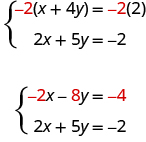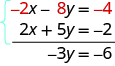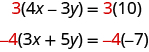# 5.3 Solve systems of equations by elimination

 Page 1 / 6
By the end of this section, you will be able to:
• Solve a system of equations by elimination
• Solve applications of systems of equations by elimination
• Choose the most convenient method to solve a system of linear equations

Before you get started, take this readiness quiz.

1. Simplify $-5\left(6-3a\right)$ .
If you missed this problem, review [link] .
2. Solve the equation $\frac{1}{3}x+\frac{5}{8}=\frac{31}{24}$ .
If you missed this problem, review [link] .

We have solved systems of linear equations by graphing and by substitution. Graphing works well when the variable coefficients are small and the solution has integer values. Substitution works well when we can easily solve one equation for one of the variables and not have too many fractions in the resulting expression.

The third method of solving systems of linear equations is called the Elimination Method. When we solved a system by substitution, we started with two equations and two variables and reduced it to one equation with one variable. This is what we’ll do with the elimination method, too, but we’ll have a different way to get there.

## Solve a system of equations by elimination

The Elimination Method is based on the Addition Property of Equality. The Addition Property of Equality says that when you add the same quantity to both sides of an equation, you still have equality. We will extend the Addition Property of Equality to say that when you add equal quantities to both sides of an equation, the results are equal.

For any expressions a , b , c , and d ,

$\begin{array}{cccc}\text{if}\hfill & \hfill a& =\hfill & b\hfill \\ \text{and}\hfill & \hfill c& =\hfill & d\hfill \\ \text{then}\hfill & \hfill a+c& =\hfill & b+d\hfill \end{array}$

To solve a system of equations by elimination, we start with both equations in standard form. Then we decide which variable will be easiest to eliminate. How do we decide? We want to have the coefficients of one variable be opposites, so that we can add the equations together and eliminate that variable.

Notice how that works when we add these two equations together:

$\begin{array}{c}3x+y=5\hfill \\ \underset{\text{_________}}{2x-y=0}\hfill \\ 5x\phantom{\rule{1.7em}{0ex}}=5\hfill \end{array}$

The y ’s add to zero and we have one equation with one variable.

Let’s try another one:

$\left\{\begin{array}{c}x+4y=2\hfill \\ 2x+5y=-2\hfill \end{array}$

This time we don’t see a variable that can be immediately eliminated if we add the equations.

But if we multiply the first equation by −2, we will make the coefficients of x opposites. We must multiply every term on both sides of the equation by −2.Now we see that the coefficients of the x terms are opposites, so x will be eliminated when we add these two equations.

Add the equations yourself—the result should be −3 y = −6. And that looks easy to solve, doesn’t it? Here is what it would look like.We’ll do one more:

$\left\{\begin{array}{c}4x-3y=10\hfill \\ 3x+5y=-7\hfill \end{array}$

It doesn’t appear that we can get the coefficients of one variable to be opposites by multiplying one of the equations by a constant, unless we use fractions. So instead, we’ll have to multiply both equations by a constant.

We can make the coefficients of x be opposites if we multiply the first equation by 3 and the second by −4, so we get 12 x and −12 x .This gives us these two new equations:

$\left\{\begin{array}{c}\phantom{\rule{1.1em}{0ex}}12x-9y=30\hfill \\ -12x-20y=28\hfill \end{array}$

$\begin{array}{c}\left\{\begin{array}{c}\phantom{\rule{1.1em}{0ex}}12x-9y=30\hfill \\ \underset{\text{_____________}}{-12x-20y=28}\hfill \end{array}\\ \hfill -29y=58\end{array}$

the x ’s are eliminated and we just have −29 y = 58.

Bruce drives his car for his job. The equation R=0.575m+42 models the relation between the amount in dollars, R, that he is reimbursed and the number of miles, m, he drives in one day. Find the amount Bruce is reimbursed on a day when he drives 220 miles.
LeBron needs 150 milliliters of a 30% solution of sulfuric acid for a lab experiment but only has access to a 25% and a 50% solution. How much of the 25% and how much of the 50% solution should he mix to make the 30% solution?
5%
Michael
hey everyone how to do algebra
Felecia answer 1.5 hours before he reaches her
I would like to solve the problem -6/2x
12x
Andrew
how
Christian
Does the x represent a number or does it need to be graphed ?
latonya
-3/x
Venugopal
-3x is correct
Atul
Arnold invested $64,000, some at 5.5% interest and the rest at 9%. How much did he invest at each rate if he received$4,500 in interest in one year?
Tickets for the community fair cost $12 for adults and$5 for children. On the first day of the fair, 312 tickets were sold for a total of $2204. How many adult tickets and how many child tickets were sold? Alpha Reply 220 gayla Three-fourths of the people at a concert are children. If there are 87 children, what is the total number of people at the concert? Tsimmuaj Reply Erica earned a total of$50,450 last year from her two jobs. The amount she earned from her job at the store was $1,250 more than four times the amount she earned from her job at the college. How much did she earn from her job at the college? Tsimmuaj Erica earned a total of$50,450 last year from her two jobs. The amount she earned from her job at the store was $1,250 more than four times the amount she earned from her job at the college. How much did she earn from her job at the college? Tsimmuaj ? Is there anything wrong with this passage I found the total sum for 2 jobs, but found why elaborate on extra If I total one week from the store *4 would = the month than the total is = x than x can't calculate 10 month of a year candido what would be wong candido 87 divided by 3 then multiply that by 4. 116 people total. Melissa the actual number that has 3 out of 4 of a whole pie candido was having a hard time finding Teddy use Matrices for the 2nd question Daniel One number is 11 less than the other number. If their sum is increased by 8, the result is 71. Find the numbers. Tsimmuaj Reply 26 + 37 = 63 + 8 = 71 gayla 26+37=63+8=71 ziad 11+52=63+8=71 Thisha how do we know the answer is correct? Thisha 23 is 11 less than 37. 23+37=63. 63+8=71. that is what the question asked for. gayla 23 +11 = 37. 23+37=63 63+8=71 Gayla by following the question. one number is 11 less than the other number 26+11=37 so 26+37=63+8=71 Gayla your answer did not fit the guidelines of the question 11 is 41 less than 52. gayla 71-8-11 =52 is this correct? Ruel let the number is 'x' and the other number is "x-11". if their sum is increased means: x+(x-11)+8 result will be 71. so x+(x-11)+8=71 2x-11+8=71 2x-3=71 2x=71+3 2x=74 1/2(2x=74)1/2 x=37 final answer tesfu just new Muwanga Amara currently sells televisions for company A at a salary of$17,000 plus a $100 commission for each television she sells. Company B offers her a position with a salary of$29,000 plus a $20 commission for each television she sells. How televisions would Amara need to sell for the options to be equal? Tsimmuaj Reply yes math Kenneth company A 13 company b 5. A 17,000+13×100=29,100 B 29,000+5×20=29,100 gayla need help with math to do tsi test Toocute me too Christian have you tried the TSI practice test ***tsipracticetest.com gayla DaMarcus and Fabian live 23 miles apart and play soccer at a park between their homes. DaMarcus rode his bike for 34 of an hour and Fabian rode his bike for 12 of an hour to get to the park. Fabian’s speed was 6 miles per hour faster than DaMarcus’s speed. Find the speed of both soccer players. gustavo Reply ? Ann DaMarcus: 16 mi/hr Fabian: 22 mi/hr Sherman Joy is preparing 20 liters of a 25% saline solution. She has only a 40% solution and a 10% solution in her lab. How many liters of the 40% solution and how many liters of the 10% solution should she mix to make the 25% solution? Wenda Reply 15 and 5 32 is 40% , & 8 is 10 % , & any 4 letters is 5%. Karen It felt that something is missing on the question like: 40% of what solution? 10% of what solution? Jhea its confusing Sparcast 3% & 2% to complete the 25% Sparcast because she already has 20 liters. Sparcast ok I was a little confused I agree 15% & 5% Sparcast 8,2 Karen Jim and Debbie earned$7200. Debbie earned \$1600 more than Jim earned. How much did they earned
5600
Gloria
1600
Gloria
Bebbie: 4,400 Jim: 2,800
Jhea
A river cruise boat sailed 80 miles down the Mississippi River for 4 hours. It took 5 hours to return. Find the rate of the cruise boat in still water and the rate of the current.
A veterinarian is enclosing a rectangular outdoor running area against his building for the dogs he cares for. He needs to maximize the area using 100 feet of fencing. The quadratic equation A=x(100−2x) gives the area, A , of the dog run for the length, x , of the building that will border the dog run. Find the length of the building that should border the dog run to give the maximum area, and then find the maximum area of the dog run.
ggfcc
MikeByBy Nick SwainByByBy Mldelatte